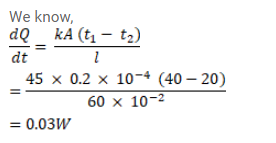# A steel frame (K = 45W/m-°C) of total length 60cm andQuestion:

A steel frame $\left(\mathrm{K}=45 \mathrm{~W} / \mathrm{m}^{\circ} \mathrm{C}\right)$ of total length $60 \mathrm{~cm}$ and cross - sectional area $0.20 \mathrm{~cm}^{2}$ forms three sides of a square. The free ends are maintained at $20^{\circ} \mathrm{C}$ and $40^{\circ} \mathrm{C}$. Find the rate of heat flow through a cross section of the frame.

Solution: